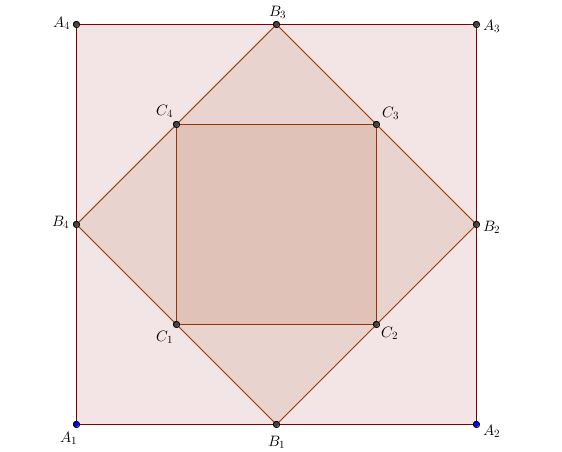# Infinite Geometric Progression

Geometry Level 2A square $B_1B_2B_3B_4$ is formed by joining the midpoints of the sides of a square $A_1A_2A_3A_4$. A third square $C_1C_2C_3C_4$ is made in the same way and the process is continued indefinitely.

If $A_1A_2 = 10 \text{ cm}$, find the sum of the areas of all the squares so formed in $\text{cm}^{2}$.

×

Problem Loading...

Note Loading...

Set Loading...